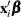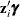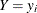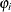OUTPUT Statement

OUTPUT <OUT=SAS-data-set> <output-options> ;

The OUTPUT statement creates a new SAS data set that contains all the variables in the input data set and, optionally, the estimates of, the expected value of the response variable, and the probability that the response variable will take on the current value or other values that you specify. In a zero-inflated model, you can additionally request that the output data set contain the estimates ofand the probability that the response is zero as a result of the zero-generating process. Except for the probability of the current value, these statistics can be computed for all observations in which the regressors are not missing, even if the response is missing. By adding observations with missing response values to the input data set, you can compute these statistics for new observations or for settings of the regressors that are not present in the data without affecting the model fit.

You can specify only one OUTPUT statement. You can specify the following OUTPUT statement options:

OUT=SAS-data-set

names the output data set.

XBETA=name

names the variable that contains estimates of.

PRED=name

names the variable that contains the predicted value of the response variable.

PROB=name

names the variable that contains the probability of the response variable taking the current value, Pr().

PROBCOUNT(value1 <value2...>)

outputs the probability of the response variable taking particular values. Each value should be a nonnegative integer. Nonintegers are rounded to the nearest integer. value can also be a list of the form X TO Y BY Z. For example, PROBCOUNT(0 1 2 TO 10 BY 2 15) requests predicted probabilities for counts 0, 1, 2, 4, 5, 6, 8, 10, and 15.

ZGAMMA=name

names the variable that contains estimates of.

PROBZERO=name

names the variable that contains the value of, the probability that the response variable will take on the value of zero as a result of the zero-generating process. It is written to the output file only if the model is zero-inflated. Note that this is not the overall probability of a zero response. That is provided by the PROBCOUNT(0) option.